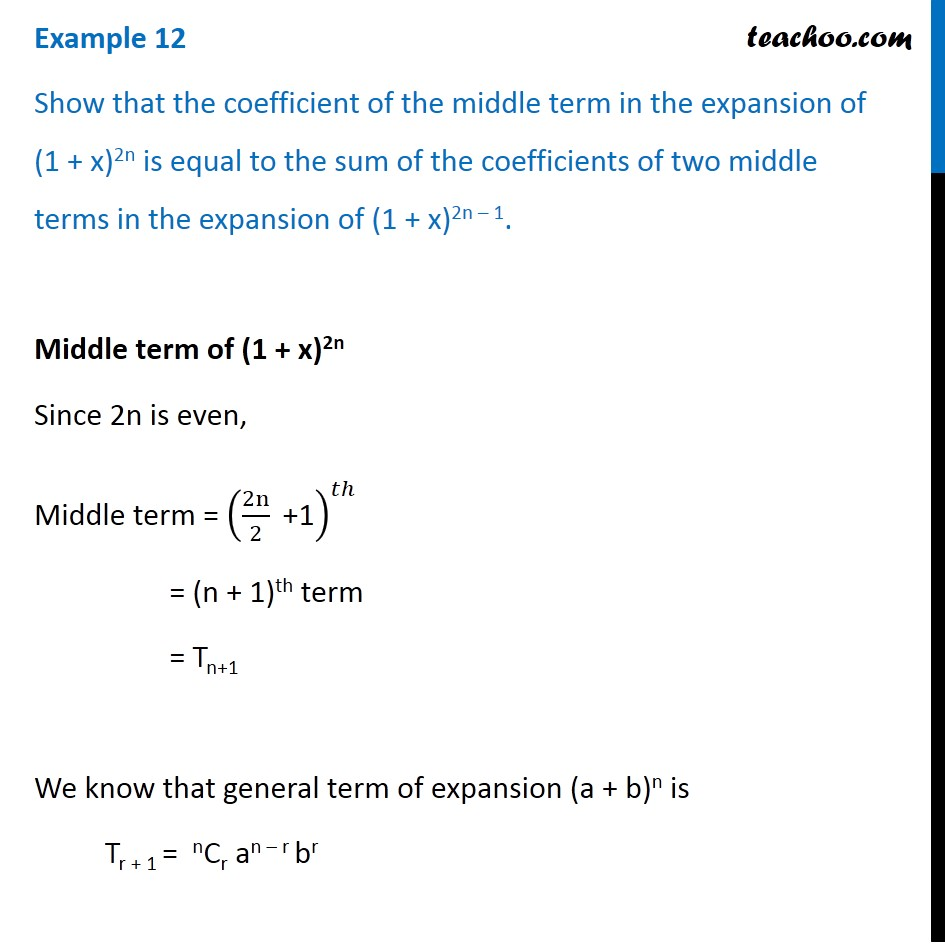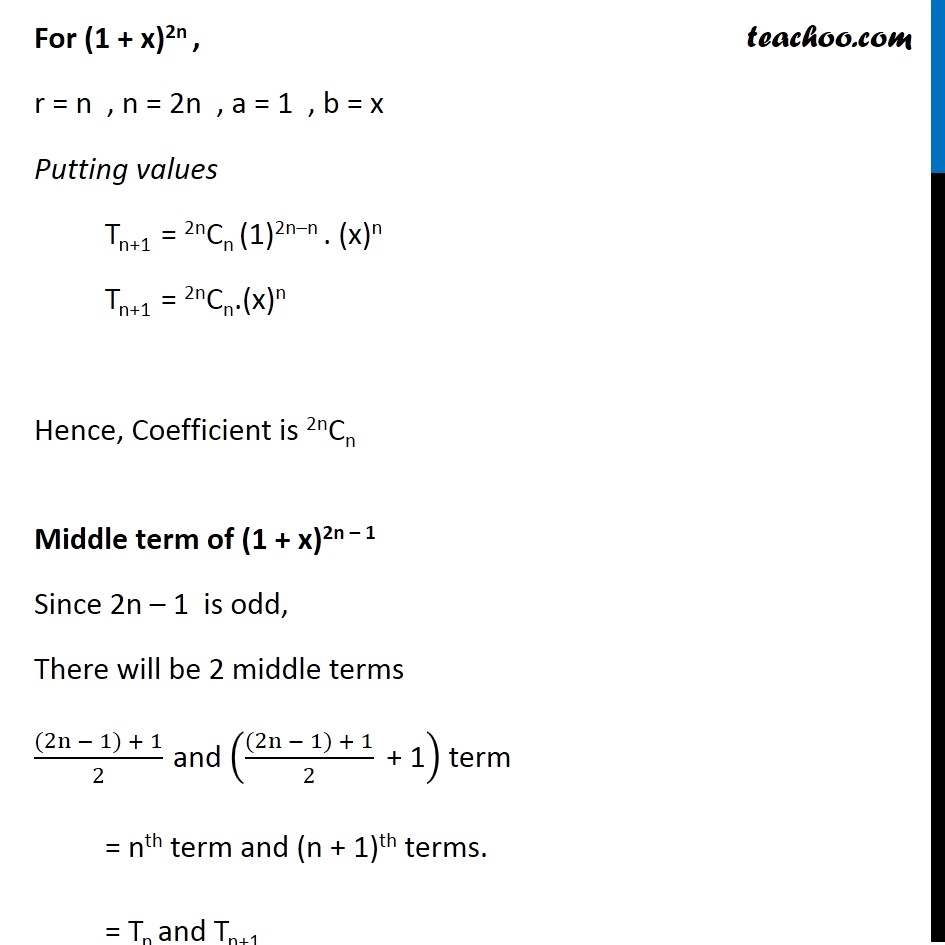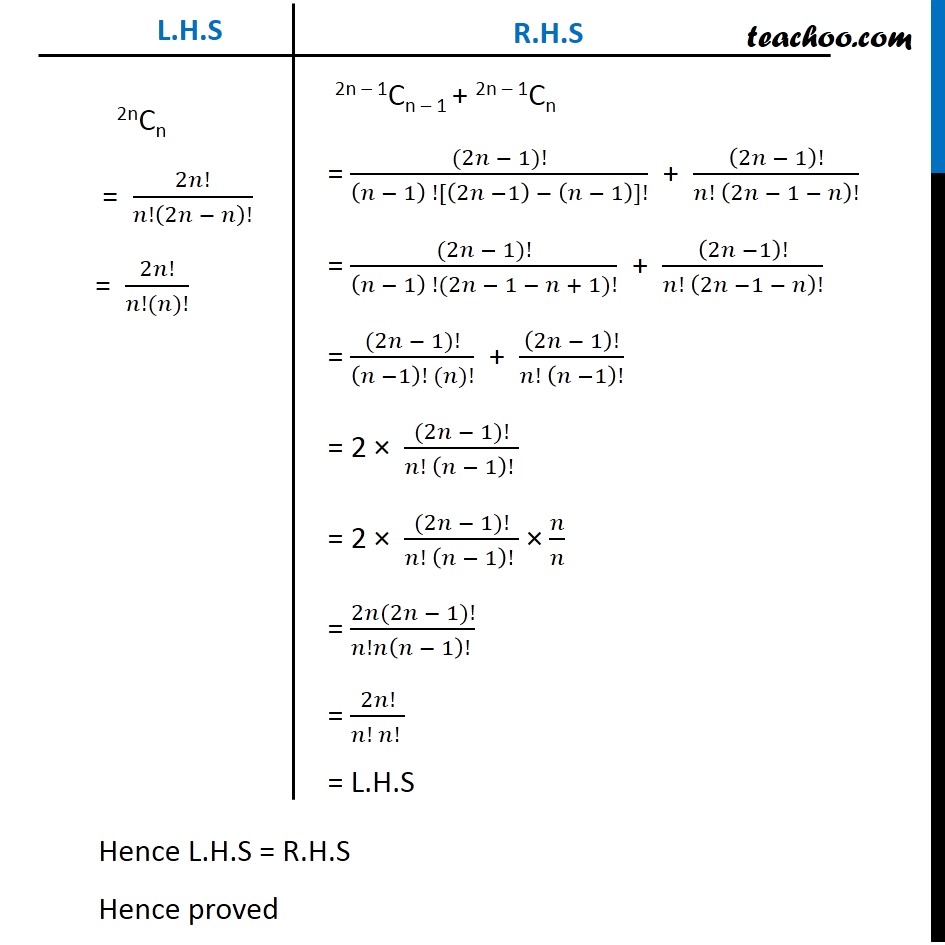1. Chapter 8 Class 11 Binomial Theorem (Deleted)
2. Serial order wise
3. Examples

Transcript

Example 12 Show that the coefficient of the middle term in the expansion of (1 + x)2n is equal to the sum of the coefficients of two middle terms in the expansion of (1 + x)2n – 1. Middle term of (1 + x)2n Since 2n is even, Middle term = (2n/2 " +1" )^𝑡ℎ = ("n + 1")th term = Tn+1 We know that general term of expansion (a + b)n is Tr + 1 = nCr an – r br For (1 + x)2n , r = n , n = 2n , a = 1 , b = x Putting values Tn+1 = 2nCn (1)2n–n . (x)n Tn+1 = 2nCn.(x)n Hence, Coefficient is 2nCn Middle term of (1 + x)2n – 1 Since 2n – 1 is odd, There will be 2 middle terms ((2n − 1) + 1)/2 and (((2n − 1) + 1)/2 " + 1" ) term = nth term and (n + 1)th terms. = Tn and Tn+1 We know that general term for expansion (a + b)n Tr+1 = n Cr an–r br For Tn in (1 + x)2n – 1 Putting r = n – 1 , n = 2n – 1 , a = 1 & b = x Tn – 1 + 1 = 2n – 1Cn – 1 . (1) (2n – 1) – (n–1) .x n – 1 Tn = 2n – 1Cn – 1 .(x)n – 1 Hence, coefficient is 2n – 1Cn – 1 Similarly, For Tn + 1 in (1 + x)2n – 1 Putting r = n , n = 2n – 1 , a = 1 & b = x Tn+1 = 2n – 1Cn . (1)2n – 1 – n . xn = 2n–1Cn . xn Hence, Coefficient is 2n–1Cn Now we have to prove Coefficient of middle term of (1+x)2n = Sum of coefficient of middle term of (1 + x)2n–1 2nCn = 2n – 1Cn – 1 + 2n – 1Cn Thus, We have to prove 2nCn = 2n – 1Cn – 1 + 2n – 1Cn 2nCn = 2𝑛!/𝑛!(2𝑛 − 𝑛)! = 2𝑛!/(𝑛!(𝑛)!) 2n – 1Cn – 1 + 2n – 1Cn = ((2𝑛 − 1)!)/((𝑛 − 1) ![(2𝑛 −1) − (𝑛 − 1)]!) + (2𝑛 − 1)!/(𝑛! (2𝑛 − 1 − 𝑛)!) = ((2𝑛 − 1)!)/((𝑛 − 1) !(2𝑛 − 1 − 𝑛 + 1)!) + (2𝑛 −1)!/(𝑛! (2𝑛 −1 − 𝑛)!) = ((2𝑛 − 1)!)/((𝑛 −1)! (𝑛)!) + (2𝑛 − 1)!/(𝑛! (𝑛 −1)!) = 2 × ((2𝑛 − 1)!)/(𝑛! (𝑛 − 1)! ) = 2 × ((2𝑛 − 1)!)/(𝑛! (𝑛 − 1)! ) × 𝑛/𝑛 = (2𝑛(2𝑛 − 1)!)/(𝑛!𝑛(𝑛 − 1)! ) = 2𝑛!/(𝑛! 𝑛! ) = L.H.S Hence L.H.S = R.H.S Hence proved

Examples

Chapter 8 Class 11 Binomial Theorem (Deleted)
Serial order wise